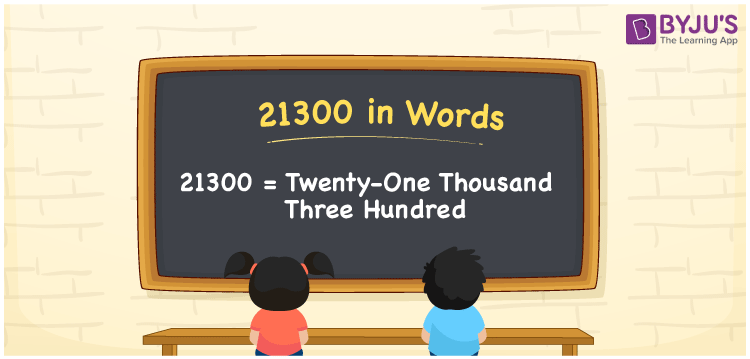# 21300 in Words

21300 in words is “Twenty-one Thousand Three Hundred”. To count the objects or to express the value, the numbers can be used. For example, if a library has 21300 books, it can be written as “A library has twenty-one thousand three hundred books. For writing the number 21300 in words, the place value table is very useful. Here is the complete explanation on how to write 21300 in words.

 21300 in Words: Twenty-one thousand three hundred. Twenty-one thousand three hundred in Numerical Form: 21300.

## 21300 in English Words## How to Write 21300 in Words?

We can employ the place value system to write the number name of 21300. The following is the place value table for the number 21300.

 Ten – Thousands Thousands Hundreds Tens Ones 2 1 3 0 0

We can write the expanded form of 21300 as follows:

= 2 × Ten thousand + 1 × Thousand + 3 × Hundred + 0 × Ten + 0 × One

= 2 × 10000 +1 × 1000 + 3 × 100

= 20000 + 1000 + 300

= 21300

= twenty-one thousand three hundred

Hence, 21300 in words is twenty-one thousand three hundred.

The number 21300 is a natural number that appears between 21299 and 21301.

21300 in words – Twenty-one thousand three hundred

Is 21300 an odd number? – No

Is 21300 an even number? – Yes

Is 21300 a perfect square number? – No

Is 21300 a perfect cube number? – No

Is 21300 a prime number? – No

Is 21300 a composite number? – Yes

## Frequently Asked Questions on 21300 in Words

Q1

### How to spell 21300?

21300 in words is twenty-one thousand three hundred.

Q2

### Simplify 21000 + 300, and express the sum in words.

Simplifying 21000 + 300, we get 21300. Hence, 21300 in words is twenty-one thousand three hundred.

Q3

### Is 21300 an even number?

Yes, 21300 is an even number.